#### Howdy, Stranger!

We are about to switch to a new forum software. Until then we have removed the registration on this forum.

# How to draw a circular line graph from data (csv)?

edited November 2015

Hi there, I'm trying to draw a weather diagram. What I got so far is the diagram for the temperature, drawn by a dot for maximum temperature and a dot for minimum temperature both connected by a line, in a nice circle. Everything drawn with the help of rotate(). This code works so far. But I want to do now is to "connect" the dots to a "circular line chart". One line for the max. Temperatur and one line for the min. temperature. I guess this cannot be done with rotate(). I tried something else (see the code at the bottom), but it doesnt work and I have no idea how to continue.

This is the working code:

```
//Array for Temperature
float[] airTempMax;
float[] airTempMin;

Table table;
int rowCount;

//coordinates for circles
float x = 40;
float y = 40;

void setup() {
background(230);
size(1100, 1100);
smooth();
noFill();
strokeWeight(1.2);
ellipseMode(CENTER);
colorMode(HSB, 60);
initializeMaxTemp();
initializeMinTemp();
println(airTempMin);

noLoop();
}

void initializeMaxTemp() {

//load the csv
table = loadTable("Wetter_Potsdam.csv", "header");

//count the rows
rowCount = table.getRowCount();

//length of array (-1 because last row is empty)
airTempMax = new float[rowCount-1];

// Array airTempMax is filled up here
for (int i = 0; i < airTempMax.length; i++) {
airTempMax[i] = table.getFloat(i, 9);
}
}

void initializeMinTemp() {

//load the csv
table = loadTable("Wetter_Potsdam.csv", "header");

//count the rows
rowCount = table.getRowCount();

//length of array (-1 because last row is empty)
airTempMin = new float[rowCount-1];

// Array airTempMax is filled up here
for (int k = 0; k < airTempMin.length; k++) {
airTempMin[k] = table.getFloat(k, 10);
}
}

// here the tempurate is drawn and every day got its own color depending on temperature
void temperature() {

for (int j = 0; j < airTempMax.length; j++) {

//color of each stroke
stroke(airTempMin[j]+40, 100, 100);

// every single line got rotated by one unit, all units equal 360 (deegree)
rotate(radians(360.0/365));
strokeWeight(0.2);
line((airTempMin[j]*4)+150, 0, (airTempMax[j]*4)+150, 0);
noStroke();
fill(airTempMin[j]+40, 100, 100);
ellipse((airTempMax[j]*4)+150,0,3,3);
ellipse((airTempMin[j]*4)+150,0,3,3);
}
}

void draw() {

//we need translate here in order to have a visual anchor to read this diagram
translate(width/2, height/2);

// here the whole circle is rotate by 240 deegree
rotate(radians(240));

strokeWeight(1);
temperature();
}

```

What I tried so far but stuck is to draw the line with vertex, but it doesnt work like I want it to.

```
int radius = 200;
float[] airTempMax;

Table table;
int rowCount;

void setup(){
size(500,500);
noFill();
noLoop();
initializeMaxTemp();
println(airTempMax);
}

void initializeMaxTemp() {

//load the csv
table = loadTable("Wetter_Potsdam.csv", "header");

//count the rows
rowCount = table.getRowCount();

//length of array (-1 because last row is empty)
airTempMax = new float[rowCount-1];

// Array airTempMax is filled up here
for (int i = 0; i < airTempMax.length; i++) {
airTempMax[i] = table.getFloat(i, 9);
}
}

void draw(){
translate(width/2,height/2);
background(204);
beginShape();
for(int deg = 0; deg < 365; deg++){
float angle = radians(deg);
float x = airTempMax[deg] + (cos(angle) * radius);
float y = 5 + (sin(angle) * radius);
vertex(x,y);
}
endShape();
}

```

If you have any ideas to solve this problem please let me know. Thank you!!

Tagged:

## Answers

• Not tested; but if you want a circular graph; then in lines 38-39 'radius' should represent the height of the graph; so this needs to be multiplied by the required height; and not added to it. It also needs to feed both the x and y position:

``````float x = cos(angle) * radius * airTempMax[deg];
float y =  sin(angle) * radius * airTempMax[deg];
``````

You can adjust the value of radius to change the scale of the overall graph...

• Thank you for your answer, it's getting closer. I've adjusted the overall size and the radius and this was the visual output.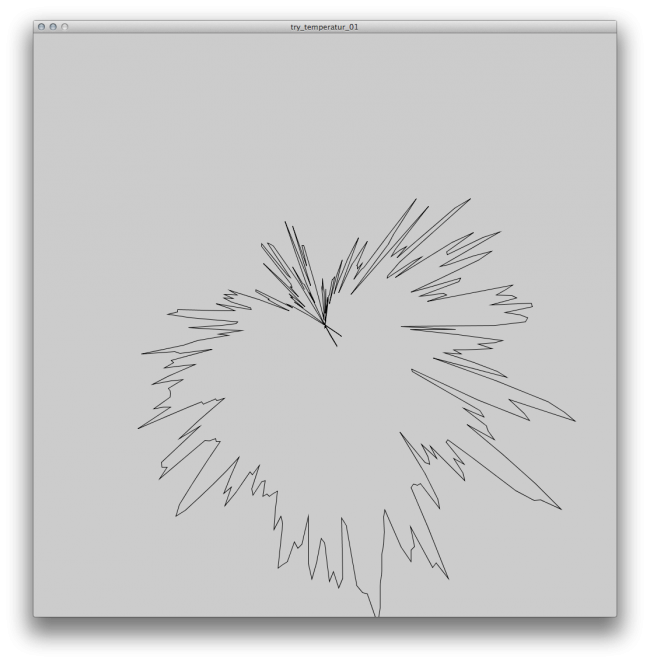Could causing the degrees which are below zero the weird look? In comparison with the »actual« graph its close but still strange. Here a picture from my first code. The outer ring shows the maximum temperature for each day.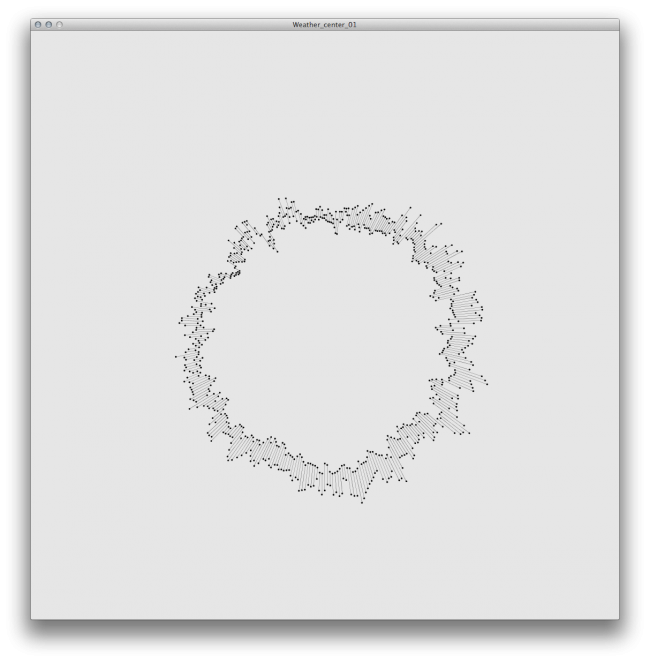This is what I changed:

```int radius = 15;
float[] airTempMax;

Table table;
int rowCount;

void setup(){
size(1000,1000);
noFill();
noLoop();
initializeMaxTemp();
println(airTempMax);
}

void initializeMaxTemp() {

//load the csv
table = loadTable("Wetter_Potsdam.csv", "header");

//count the rows
rowCount = table.getRowCount();

//length of array (-1 because last row is empty)
airTempMax = new float[rowCount-1];

// Array airTempMax is filled up here
for (int i = 0; i < airTempMax.length; i++) {
airTempMax[i] = table.getFloat(i, 9);
}
}

void draw(){
translate(width/2,height/2);
//the rotation is for having January 1st at the top
rotate(radians(240));
background(204);
beginShape();
for(int deg = 0; deg < 365; deg++){
float angle = radians(deg);
float x = cos(angle) * radius * airTempMax[deg];
float y = sin(angle) * radius * airTempMax[deg];
vertex(x,y);
}
endShape(CLOSE);
}
```
• Oops.. Try this instead:

``````float x = cos(angle) * (radius + airTempMax[deg]);
float y = sin(angle) * (radius + airTempMax[deg]);
``````

The problem with my original was that multiplying radius and airTempMax massively exaggerates the difference between values and doesn't account for minus values. When using addition, as above, 'radius' will represent the zero scale. If you do still need to apply scaling you could multiply airTempMax by a separate variable.

• Thank you once again. But I changed the vertex to an ellipse to verify if it works. It does somehow but there is still one problem left. It seems like the ends are overlap at the top. I took two screenshot, one with the code you provided (which is pretty close to solve this problem) and one with my »Test circle«, from the very first code I've tried and posted here. I set the variable deg to 365/360 but it didnt solve the problem.

the black circle is zero degree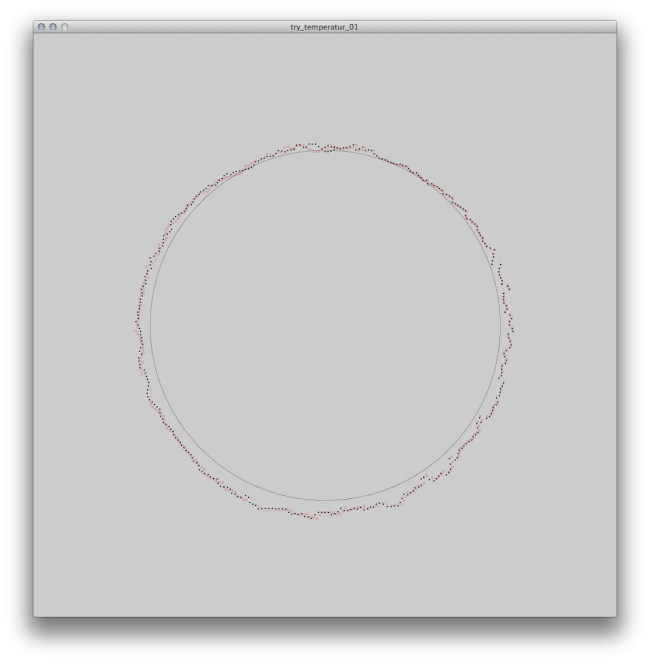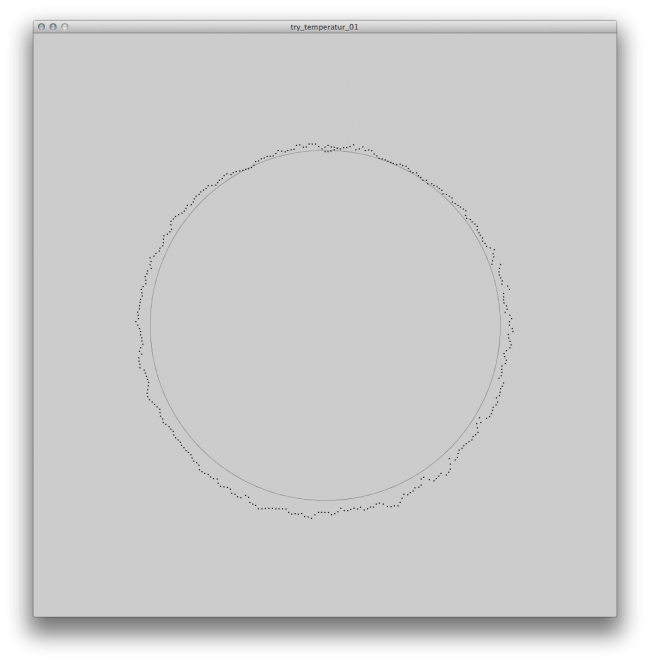Here my current code

```int radius = 300;
float[] airTempMax;

Table table;
int rowCount;

void setup() {
size(1000, 1000);
noFill();
noLoop();
ellipseMode(CENTER);
initializeMaxTemp();
}

void initializeMaxTemp() {

//load the csv
table = loadTable("Wetter_Potsdam.csv", "header");

//count the rows
rowCount = table.getRowCount();

//length of array (-1 because last row is empty)
airTempMax = new float[rowCount-1];

// Array airTempMax is filled up here
for (int i = 0; i < airTempMax.length; i++) {
airTempMax[i] = table.getFloat(i, 9);
}
}

void tempMax() {
translate(width/2, height/2);

//the rotation is for having January 1st at the top
rotate(radians(270));
background(204);
//beginShape(POINTS);
for (int deg = 0; deg < airTempMax.length; deg++) {
float angle = radians(deg);
float x = cos(angle) * (radius + airTempMax[deg]);
float y = sin(angle) * (radius + airTempMax[deg]);
ellipse(x, y, 1, 1);
println(airTempMax[deg]);
}
//endShape(CLOSE);
}

void tempMaxProof() {
for (int j = 0; j < airTempMax.length; j++) {

// every single line got rotated by one unit, all units equal 360 (deegree)
rotate(radians(360.0/365));
stroke(200, 0, 0);
ellipse((airTempMax[j]+300), 0, 3, 3);

}
}

void draw() {

tempMax();

strokeWeight(0.3);
ellipse(0, 0, 600, 600);
tempMaxProof();
}

```
• It's hard to say without having the source data to test with :/

I wonder if there's a floating point inaccuracy issue here. In your overlapped image the two versions start off very closely matching and the further you go round the less well they line up.

Hard to know the cause. It's almost as though `radians(360.0/365)` is returning a different value. Have you checked that airTempMax.length is the same in both versions? Has anything else changed?

• Nothing at the data set has changed. The length is still the same, 365 entries in the array airTempMax. This is the data set (Dropbox Link, csv file)

• Could be the problem the length of the array with 365, which is 5 more than 360? I'm asking because I tried the `for (int deg = 0; deg < 360; deg++)` and nothing overlapped. I tried it with 361 and it's overlapping again. So I have tried this, which looks like it draws all the points at the right position but in a »beautiful« straight line. So I'm actually looking for a solution to tell processing to go over the array but only ad 0.9 something degree to the circle, I guess.

```int radius = 300;
float[] airTempMax;
int k = 0;

Table table;
int rowCount;

void setup() {
size(1000, 1000);
noFill();
noLoop();
ellipseMode(CENTER);
initializeMaxTemp();
println(airTempMax);
}

void initializeMaxTemp() {

//load the csv
table = loadTable("Wetter_Potsdam.csv", "header");

//count the rows
rowCount = table.getRowCount();

//length of array (-1 because last row is empty)
airTempMax = new float[rowCount-1];

// Array airTempMax is filled up here
for (int i = 0; i < airTempMax.length; i++) {
airTempMax[i] = table.getFloat(i, 9);
}
}

void tempMax() {
translate(width/2, height/2);

//the rotation is for having January 1st at the top
rotate(radians(270));
background(204);
//beginShape(POINTS);
for (float deg = 0; deg < airTempMax.length; deg = deg + 0.98630136986301) {
k = 0;
k++;
float angle = radians(deg);
float x = cos(angle) * (radius + airTempMax[k]);
float y = sin(angle) * (radius + airTempMax[k]);
ellipse(x, y, 1, 1);

}
//endShape(CLOSE);
}

void tempMaxProof() {
for (int j = 0; j < airTempMax.length; j++) {

// every single line got rotated by one unit, all units equal 360 (deegree)
rotate(radians(360.0/365));
stroke(200, 0, 0);
ellipse((airTempMax[j]+300), 0, 3, 3);

}
}

void draw() {

tempMax();

strokeWeight(0.3);
ellipse(0, 0, 600, 600);
tempMaxProof();
}
```
• Picture to my previous comment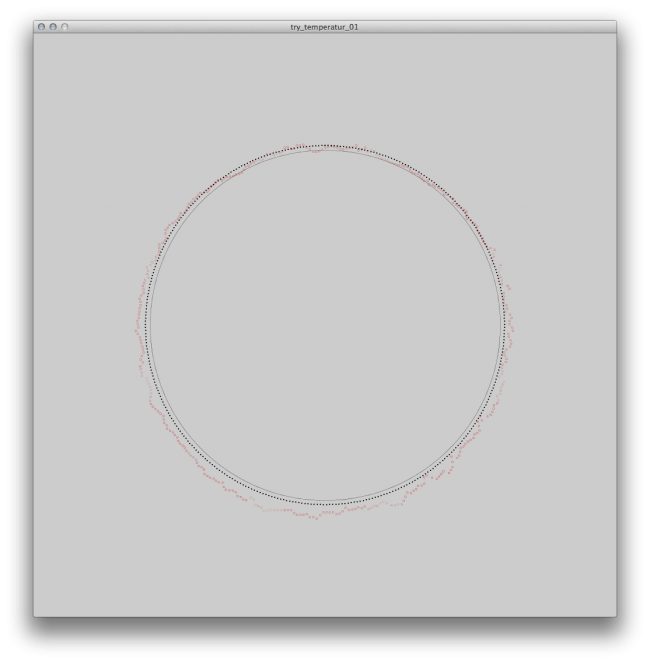• OK, it just dawned on me what your problem is and it's demonstrated by this:

``````for (float deg = 0; deg < airTempMax.length; deg = deg + 0.98630136986301) {
// snip
}
``````

You're doing things backwards here! Forget about degrees in your loop for the moment. The key thing is to iterate through all items in your data:

``````for (int i = 0; i < airTempMax.length; i++) {
// snip
}
``````

The problem you're having is that your data has more points than a full circle... but you already know how to calculate the required angle step (you're using it in rotate() in tempMaxProof()); so you can just store that value before the loop. Then just multiply this by `i` to get the angle to draw to in the current loop iteration:

``````// will work with *any* length of airTempMax (except 0!):
float stepAngle = radians(360.0/airTempMax.length);

for (int i = 0; i < airTempMax.length; i++) {

float angle = i * stepAngle;
float x = cos(angle) * (radius + airTempMax[i]);
float y = sin(angle) * (radius + airTempMax[i]);
ellipse(x, y, 1, 1);

}
``````

I haven't tested this (I do mostly p5js stuff these days); but the principle should be sound :)

• BTW: you don't need the `rotate(radians(270))` to get the desired start position. You could do:

``````float angle = startAngleInRadians + (i * stepAngle);
``````

Set the value of startAngleInRadians before the loop...

This change is a matter of personal taste though: I prefer to avoid rotate()

• Thank you. Also for the good advise how to get rid of rotate().

Sign In or Register to comment.